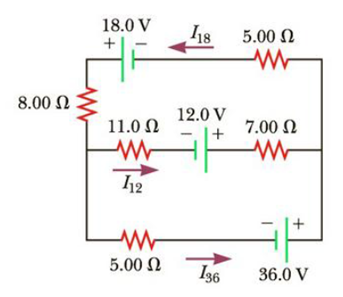Chapter 18, Problem 30P

Chapter
Section
Textbook Problem

For the circuit shown in Figure P18.30, use Kirchhoff’s rules to obtain equations for (a) the upper loop, (b) the lower loop, and (c) the node on the left side. In Figure P18.29 each case suppresses units for clarity and simplify, combining like terms. (d) Solve the node equation for I36. (e) Using the equation found in (d), eliminate I36 from the equation found in part (b). (f) Solve the equations found in part (a) and part (e) simultaneously for the two unknowns for I18 and I12, respectively. (g) Substitute the answers found in part (f) into the node equation found in part (d), solving for I36. (h) What is the significance of the negative answer for I12?Figure P18.30

(a)

To determine

The equation for the upper loop by using Kirchhoff’s rule

Explanation

Given Info:

Resistance R1 is 8.00Ω Resistance R2 is 5.00Ω, Resistance R3 is 11.0Ω, Resistance R4 is 7.00Ω, Resistance R5 is 5.00Ω, Voltage ε1 is 18.0V, and Voltage ε2 is 12.0V.

Formula to calculate the equation for upper loop is by Kirchhoff’s voltage law

ε1+ε2(R1+R2)I18(R3+R4)I12=0

Substitute 8.00Ω, for R1, 11.0Ω,for R2, 5.00Ω for R3, 7.00Ω for R4 18.0V for ε112

(b)

To determine

The equation for the lower loop by using Kirchhoff’s rule.

(c)

To determine

The equation of node on the left side by using Kirchhoff’s rule.

(d)

To determine

The equation of node for current I36 by using Kirchhoff’s rule.

(e)

To determine

The equation after eliminating the current I36 from the lower loop equation by using Kirchhoff’s rule.

(f)

To determine

To determine the values of the current I18.and I12

(g)

To determine

To determine the values of the current I36

(h)

To determine

To significance of the negative answer for I12

Still sussing out bartleby?

Check out a sample textbook solution.

See a sample solution

The Solution to Your Study Problems

Bartleby provides explanations to thousands of textbook problems written by our experts, many with advanced degrees!

Get Started